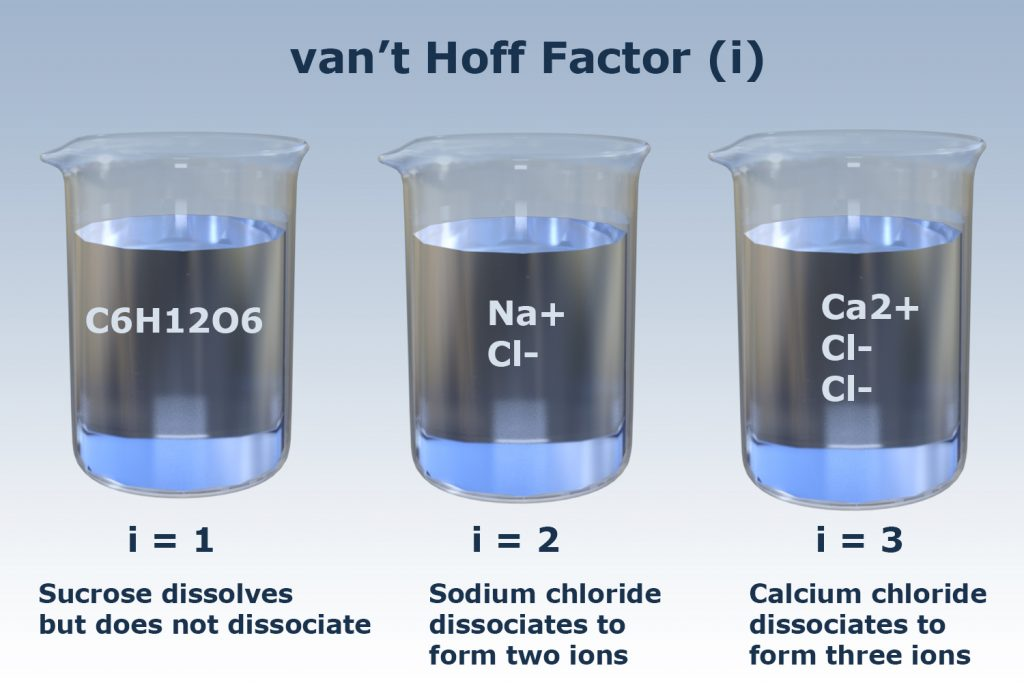Sarah Taylor

5-PS1-1

# Electrolytes and Colligative Factors Study Guide

Van’t Hoff factor can be defined as the ratio between the concentration of particles obtained when the substance is dissolved to the concentration of that substance when calculated from its mass.

# Introduction

Have you seen the behavior of different solutions? Has it ever struck you how these solutions behave like this?

Well, all the things that you see around you happen due to some reason. If you check out the electrolytic solution, there are two rods present — one rod attracts the negative ions, and another attracts the positive ions. This occurred because there is an electromagnetic force generated within the solution.

Hence, to find out the behavior and different factors affecting it, the Van’t Hoff factor is used. Now, what is Van’t Hoff's factor?

## VAN’T HOFF FACTOR

The Van't Hoff factor can be defined as the ratio between colligative property and theoretical concentrations. It is the ratio of two concentrations of particles that are formed when dissolved in a solvent by mass. It is denoted by “i”.

For an example of the Van’t Hoff factor, when you dissolve plain particles in water, the Van’t Hoff Factor, i is 1. But if you dissolve an ionic particle or compound in water, the Van't Hoff factor becomes equal to the total amount of ions present in the solution.

Hence, the greater the number of charges present, the greater will be the Van’t Hoff factor.Source

## VAN’T HOFF FACTOR EQUATION

The formula of Van’t Hoff factor is: i = 𝞪n+(1 - 𝞪)

Where, i = Van’t Hoff Factor 𝞪 = Degree of dissociation n = number of ions formed from one formula substance of the entity

## ASSOCIATION & DISSOCIATION

Association is the union of two or more particles to form one unit. An example of the association of two particles is water; as it is the biggest associated compound, it can associate with almost all particles as things will get dissolved into it.

On the other hand, dissociation refers to the separation of a molecule into numerous ionic units. For example, sodium chloride (NaCl), when dissolved in water, separates into Na and Cl– ions.

## FAQS:

1. How do you find the ionization factor?

Ionization factor can be found by using the formula i = 𝞪n+(1 - 𝞪)

2. What is the Van't Hoff factor? What is the significance of it?

The van’t Hoff factors can be defined as the ratio between the concentration of particles dissolved to the theoretical concentration. The Van't Hoff factor offers an understanding of the effect of solutes on the colligative properties of solutions.

3. How does Van't Hoff factor relate to ionization?

Van’t Hoff factor is directly related or proportional to the degree of dissociation of weak electrons. Hence, if the Van’t Hoff factor increases, so do the degree of ionization.

4. What do you understand about the degree of ionization?

The degree of ionization also termed as ionization yield, denotes the proportion of unbiased particles, like the one in gas or aqueous solution, to those that are ionized. For electrolytes, it could be comprehended as a capability of acid or base to ionize itself.

We hope you enjoyed studying this lesson and learned something cool about Electrolytes and Colligative Factors! Join our Discord community to get any questions you may have answered and to engage with other students just like you! Don't forget to download our App to experience our fun VR classrooms - we promise it makes studying much more fun!😎

## SOURCES:

1. Colligative Properties:https://www.ck12.org/section/colligative-properties/.Accessed 7th March 2022.
2. Solutions of Electrolytes: https://chem.libretexts.org/Bookshelves/General_Chemistry/Map%3A_General_Chemistry_(Petrucci_et_al.)/13%3A_Solutions_and_their_Physical_Properties/13.09%3A_Solutions_of_Electrolytes. Accessed 7th March 2022.